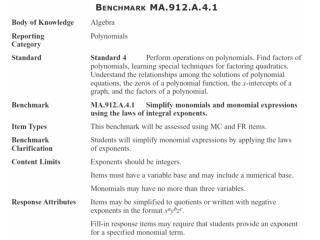Download PresentationMA.912.A.4.1: Simplify monomials and monomial expressions using the laws of integral exponents .

# MA.912.A.4.1: Simplify monomials and monomial expressions using the laws of integral exponents . - PowerPoint PPT Presentation

Algebra 1 Mini-Lessons. MA.912.A.4.1: Simplify monomials and monomial expressions using the laws of integral exponents . Algebra 1 Mini-Lessons. MA.912.A.4.1: Simplify monomials and monomial expressions using the laws of integral exponents . Algebra 1 Mini-Lessons.I am the owner, or an agent authorized to act on behalf of the owner, of the copyrighted work described.
Download Presentation## MA.912.A.4.1: Simplify monomials and monomial expressions using the laws of integral exponents .

Download Policy: Content on the Website is provided to you AS IS for your information and personal use and may not be sold / licensed / shared on other websites without getting consent from its author.While downloading, if for some reason you are not able to download a presentation, the publisher may have deleted the file from their server.

- - - - - - - - - - - - - - - - - - - - - - - - - - E N D - - - - - - - - - - - - - - - - - - - - - - - - - -
Presentation Transcript

1. Algebra 1 Mini-Lessons MA.912.A.4.1: Simplify monomials and monomial expressions using the laws of integral exponents.

2. Algebra 1 Mini-Lessons MA.912.A.4.1: Simplify monomials and monomial expressions using the laws of integral exponents.

3. Algebra 1 Mini-Lessons MA.912.A.4.1: Simplify monomials and monomial expressions using the laws of integral exponents.

4. Algebra 1 Mini-Lessons Juan simplified the following expression: His final answer was in the form bn. If he simplified the expression correctly, what is the value of n, the exponent on b? A.) 1 B.) 2 C.) 3 D.) 4 MA.912.A.4.1: Simplify monomials and monomial expressions using the laws of integral exponents.

5. Algebra 1 Mini-Lessons Todd simplified the following expression: (x8y−2)(x4y5) His final answer was in the form xmyn. If he simplified the expression correctly, what is the value of n, the exponent on y? A.) 1 B.) 2 C.) 3 D.) 4 MA.912.A.4.1: Simplify monomials and monomial expressions using the laws of integral exponents.